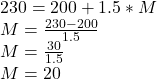Question

1. My dog Wyatt is growing at a rate of 1.5 ounces per month. He is currently 200 ounces. a) Write an expression that represents his change in weight per month, using ​M​ to

represent the amount of months.

b) Write an expression that represents his total weight after any number of months using ​M

to represent the number of months

c) Write an equation using the expression from question “b” that you could solve for ​M​ to

find how many months it will take for Wyatt to grow to a total weight of 230 ounces.

d) Solve the equation. How many months will it take for Wyatt to grow to a total weight of

230 ounces?

1.Calantha

a) Change in months after M months = 1.5 ounces per month * M

b) Weight after M months = 200 onces + 1.5 ounces per month * M

c) 20 months

d) 20 months

Step-by-step explanation:

Let M represent the number of months.

So,

a) Change in months after M months = 1.5 ounces per month * M

b) Weight after M months = 200 onces + 1.5 ounces per month * M

c)d) 20 months